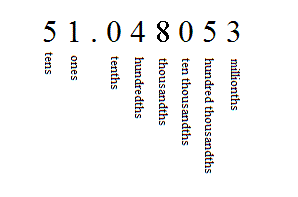# Rounding Decimals

We can round decimals to a certain accuracy or number of decimal places. This is used to make calculation easier to do and results easier to understand, when exact values are not too important.

First, you'll need to remember your place values:To round a number to the nearest tenth , look at the next place value to the right (the hundredths). If it's $4$ or less, just remove all the digits to the right. If it's $5$ or greater, add $1$ to the digit in the tenths place, and then remove all the digits to the right.

(In the example above, the hundredths digit is a $4$ , so you would get $51.0$ .)

To round a number to the nearest hundredth , look at the next place value to the right (the thousandths this time). Same deal: If it's $4$ or less, just remove all the digits to the right. If it's $5$ or greater, add $1$ to the digit in the hundredths place, and then remove all the digits to the right.

(In the example above, the thousandths digit is an $8$ , so you would get $51.05$ .)

In general, to round to a certain place value, look at the digit to the right of that place value and make a decision.

Example:

$5.1837$ to the nearest hundredth would be $5.18$ (round down since $3<5$ ),

but to the nearest thousandth, it is $5.184$ (round up because $7\ge 5$ ).

Order matters when calculating and rounding (vs. rounding then calculating):

$3.7+2.6\to 4+3\to 7$ (rounding first to nearest whole number , then adding)

$3.7+2.6\to 6.3\to 6$ (adding first and then rounding at the end.)

Which is correct? They both are. Which one you choose to use, depends upon your purpose. The second one is more accurate, because it's closer to the real answer ( $6.3$ ). In general, it's more accurate to calculate first and then round. However, sometimes if you're doing mental math and you need a quick and very approximate answer, it's easier to round and then calculate.